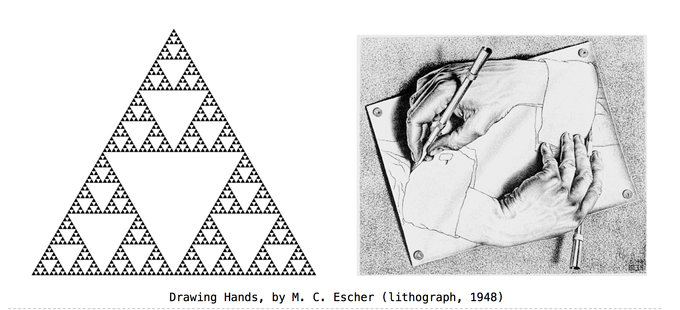# 分享 关于递归

yfractal · 2014年05月08日 · 最后由 leozwa 回复于 2014年05月09日 · 3236 次阅读1. 递归有用吗？ 觉得 web 开发里面递归并不多。 算法种似乎有很多递归（对算法了解的不多），比如树，图，链表。觉得理解了递归，这些就比较容易理解了。 递归能写出很舒服的程序，比如说 小清新

2. 如何理解递归？

``````#+BEGIN_SRC ruby
def fact(n)
if n == 1
return 1
end
return n * fact(n-1)
end
#+END_SRC
``````
3. 求证最基本的情况是否成立

4. 把 fact(n-1) 当成一种功能的抽象

5. 假设 fact(n-1) 是正确的

6. 求证 fact(n) 是否正确，在假设 f(n-1) 正确的前提下

....

1. Verify the base case.
2. Treat fact(n-1) as a functional abstraction!
3. Assume that fact(n-1) is correct.
4. Verify that fact(n) is correct, assuming that fact(n-1) correct.

``````#+BEGIN_SRC ruby
# [fib数列](http://zh.wikipedia.org/zh-cn/%E6%96%90%E6%B3%A2%E9%82%A3%E5%A5%91%E6%95%B0%E5%88%97)
nth_fib(1) == 1
nth_fib(2) == 1
nth_fib(3) == 2
nth_fib(10) == 55

# 判断一个字符串是否为回文
huiwen("a") == true
huiwen("abba") == true
huiwen("abcba") == true
huiwen("abca") == false

# count_change
# 假设有不同种的硬币比如1元，2元，5元
# 然后给出一个钱数，比如30,求有多少种找钱的可能

coin_kinds=[50, 25, 10, 5, 1]
# Return the number of ways to change amount a using coin kinds.
count_change(30) == 18
count_change(5)
count_change(1) == 1
count_change(0) == 1
#+END_SRC
``````